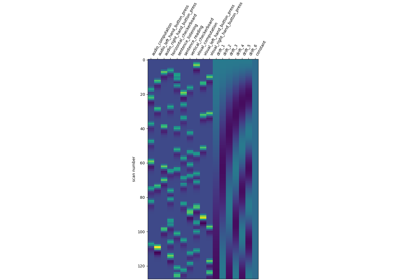Note

This page is a reference documentation. It only explains the function signature, and not how to use it. Please refer to the user guide for the big picture.

# nilearn.image.high_variance_confounds#

Return confounds extracted from input signals with highest variance.

Parameters:
imgsNiimg-like object

4D image. See Input and output: neuroimaging data representation.

If not provided, all voxels are used. If provided, confounds are extracted from voxels inside the mask. See Input and output: neuroimaging data representation.

n_confounds`int`, optional

Number of confounds to return. Default=5.

percentile`float`, optional

Highest-variance signals percentile to keep before computing the singular value decomposition, 0. <= percentile <= 100. mask_img.sum() * percentile / 100 must be greater than n_confounds. Default=2.

detrend`bool`, optional

If True, detrend signals before processing. Default=True.

Returns:
`numpy.ndarray`

Highest variance confounds. Shape: (number_of_scans, n_confounds).

Notes

This method is related to what has been published in the literature as ‘CompCor’ (Behzadi NeuroImage 2007).

The implemented algorithm does the following:

• Computes the sum of squares for each signal (no mean removal).

• Keeps a given percentile of signals with highest variance (percentile).

• Computes an SVD of the extracted signals.

• Returns a given number (n_confounds) of signals from the SVD with highest singular values.

## Examples using `nilearn.image.high_variance_confounds`#Understanding parameters of the first-level model

Understanding parameters of the first-level model